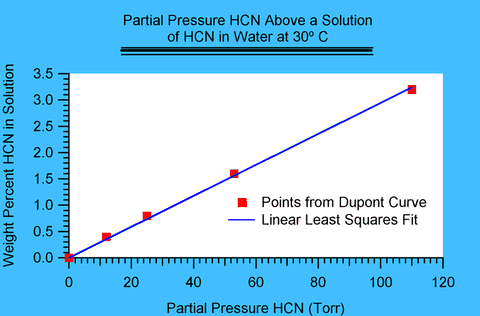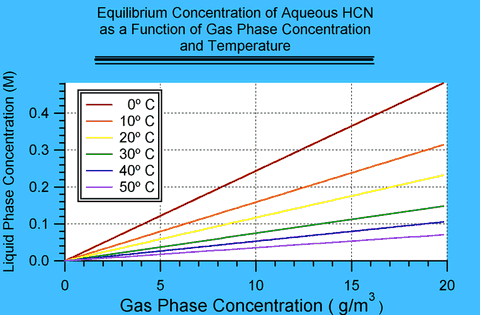## Appendix IAbsorption by Water and Henry's Law

Page 32 of DuPont's Hydrogen Cyanide: Properties, Uses, Storage and Handling 1 contains a plot of the partial pressure of HCN above aqueous solutions of HCN at various concentrations and temperature. These values are equilibrium values. That means that at these concentrations the rate of HCN in the gas phase becoming absorbed into the solution is exactly balanced by the rate of HCN leaving solution into the gas phase.

Because the plot shows equilibrium values, it contains implicitly the value of partition coefficients, i.e., it is possible to obtain the equilibrium concentration of HCN in solution in water exposed to HCN in the gas phase as a given concentration and temperature. This appendix extracts those values. In DuPont's plot liquid phase concentration is expressed in weight percent and gas phase concentration in millimeters of mercury (also known as Torr); this appendix derives relationships in terms of molarity (M) and grams per cubic meter (g/m3).

These values are equilibrium values, which means that they are an upper limit to the concentration that may be found in water exposed to HCN. How fast such equilibrium establishes itself is a question of kinetics and is a much more difficult problem.

By reading the values for a given temperature from the plot, one can construct a plot of the weight percent HCN in water as a function of gas phase concentration in Torr. The relationship is linear in the region of interest; so intermediate values can be found by fitting the points with a least-squares linear regression. At 0 Torr, the concentration in water should be 0%; therefore the fits have only one free parameter, the slope. This linear relationship is known as Henry's Law and the slope can be identified with the Henry's Law constant.

### Figure I.1Figure I.1 shows such a plot for a temperature of 30 degrees Celsius (° C). The points have been read by eye from DuPont's plot. Notice that the fit to a line is excellent and is likely to average out slight deviations in the estimates of values in DuPont's plot. The slope in this case was found to be 0.029 percent/Torr.### Temperature Dependence

Similar plots were made for 0, 10, 20, 30, 40, and 50 degrees. The values of the slope were:

Table I.1: Slope of the Gas-Liquid Partition
as a Function of Temperature.
Temperature
° CSlope
percent/Torr
00.105
10 0.066
20 0.047
30 0.029
40 0.020
50 0.013

### Unit Conversion

The problem is now essentially solved except for unit conversions. DuPont's liquid phase concentration is expressed in weight percent, and I wish to express that value in molarity (M). The first step is to calculate what volume of water contains 1 mole of HCN. The molar mass of HCN is 27.03 g. The mass of water (MH2O) can be expressed as:

(MH2O) = (100/C -1) x 27.03

where C is the concentration in weight percent HCN. The density of water is 1.0 g/mL and will be treated here as independent from temperature. HCN density as a function of temperature was found by a linear extrapolation of the densities found on page 2 of the same DuPont document. The fit in g/mL yielded:

pHCN=0.715-0.00133 x T

where T is expressed in degrees Celsius (° C), and pHCN is the density of HCN. The volume of solution that contains 1 mole of HCN can now be calculated, if one neglects the small effect of mixing on changing the volume. Figure 11 on page 31 of DuPont's handbook displays the specific gravity of HCN solutions as a function of the weight percent HCN. Inspection of this figure is sufficient to show that neglect of the volume of mixing is warranted. The volume of solution in milliliters (mL) that contains 1 mole HCN is therefore:

V= 27.03/pHCN + MH2O/1.0

I convert to molar concentration:

[HCN] = 1000/V

The gas phase concentrations of in the gas chamber were in the range of 8-16 g/m3. I therefore chose an array from 1-20 g/m3 converted that array to Torr and calculated from the above relationships the equilibrium water concentration at the given Temperatures. Conversion from g/m3 is straightforward:

P=R x T x (C/27.03) x (760/101325)

Here P is the partial pressure of HCN in Torr. R is the universal gas constant (8.31441 m3Pa/mol K (SI units!). T is the temperature in Kelvin (273.15 plus the temperature in Celsius); C is the concentration of HCN expressed in g/m3; 27.03 is the molar mass of HCN in grams (not SI units, but grams cancel); there are 760 Torr in an atmosphere and 101,325 Pascals (Pa).

### Results

The results of these calculations are shown if Figure I.2. The temperatures in the gas chambers were most likely between 20 and 40 ° C, but even if they got down to 10 ° C, and even if the higher concentrations reported were used, the equilibrium concentration of HCN is on the order of 0.1-0.2 M. In other words, these are the maximum concentrations that could be achieved. More likely, the concentration was limited by kinetics and never reached equilibrium.

### Figure I.2